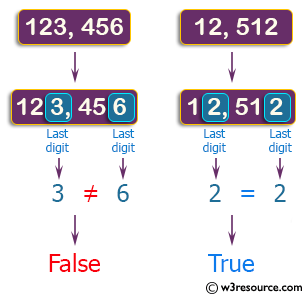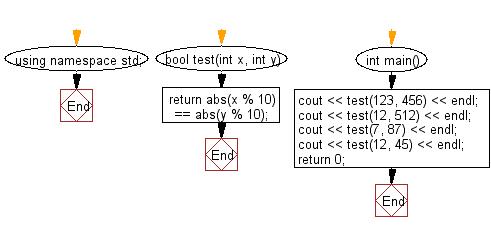﻿ C++ Exercises: Check if two given non-negative integers have the same last digit - w3resource# C++ Exercises: Check if two given non-negative integers have the same last digit

## C++ Basic Algorithm: Exercise-23 with Solution

Write a C++ program to check if two given non-negative integers have the same last digit.

Sample Solution:

C++ Code :

``````#include <iostream>
using namespace std;

bool test(int x, int y)
{
return abs(x % 10) == abs(y % 10);
}

int main()
{
cout << test(123, 456) << endl;
cout << test(12, 512) << endl;
cout << test(7, 87) << endl;
cout << test(12, 45) << endl;
return 0;
}
``````

Sample Output:

```0
1
1
0
```

Pictorial Presentation:Flowchart:C++ Code Editor:

Contribute your code and comments through Disqus.

What is the difficulty level of this exercise?

﻿